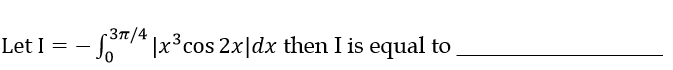156 viewsTo open the absolute value symbol, you need to decide for which $x,$ $x^3 \cos2x$ is positive or negative in the given range of $x$. Sign of $x^3 \cos2x$ is decided by the sign of $\cos 2x$ because $x^3$ is always positive for $0 \leq x \leq \frac{3 \pi}{4}.$

Here, $\cos 2x$ is positive for $0 \leq 2x \leq \frac{\pi}{2}$ or, $0 \leq x \leq \frac{\pi}{4}$ and

$\cos 2x$ is negative for $\frac{\pi}{2} \leq 2x \leq \frac{3\pi}{2}$ or, $\frac{\pi}{4} \leq x \leq \frac{3\pi}{4}$

$I = – \left(\int_{0}^{\frac{\pi}{4}}x^3 \cos 2x \ dx \ - \int_{\frac{\pi}{4}}^{\frac{3\pi}{4}}x^3 \cos 2x \ dx \right)$

You can easily compute the value of $\int x^3 \cos 2x \ dx$ which is $\frac{x^3}{2} \sin 2x + \frac{3x^2}{4}\cos 2x - \frac{3x}{4} \sin 2x - \frac{3}{8} \cos 2x$.

So, the answer is $I = \ \approx -4.4$

@ankitgupta.1729 Sir, Even I am getting it $≈ -4.4$.

1
101 views